Question

# Which of the following statements regarding the Hardy-Weinberg Equation is TRUE? Answers: It can only be u...

Which of the following statements regarding the Hardy-Weinberg Equation is TRUE?

 Answers: It can only be used when allele and genotype frequencies remain constant across generations in a population It can only be used when allele and genotype frequencies change across generations in a population It has no assumptions It mathematically shows how recessive alleles in a population decrease over time It reveals when allele frequencies change over time in a population

Assuming Hardy-Weinberg Equilibrium, what percentage of individuals in a population are carriers of Tay-Sachs disease if p = 0.45?

 Answers: 0.09% 21% 42% 49.5% 91%

Two populations are completely isolated by a mountain range, but the environments on both sides of the range are exactly the same. Which of the following microevolutionary processes explains why the allele frequencies for the two populations would be different given enough time of isolation from each other?

 Answers: Genetic Drift High levels of Gene Flow Mutation Natural Selection Random Mating

If the frequency of an X-linked recessive disorder is 1 in 50 births, what is the frequency of affected males in the population?

 Answers: 1 in 50 births 1 in 100 births 1 in 250 births 1 in 2,500 births 1 in 5,000 births

An evolutionary tree showing the relationships between past and present primates indicates which of the following?

 Answers: A species of Australopithecus turned into Chimpanzees and Humans Common ancestors can be identified by moving to more recent times on an evolutionary tree Humans share a common ancestor with the great apes Lucy is the ancestor that gave rise to Neanderthals and Humans Orangutans are more closely related to Chimpanzees than they are to Gorillas

Which of the following most accurately defines what a gene pool is?

 Answers: All of the possible genotype frequencies for a given population The change in allele frequencies over generations of time The different types of potential alleles that a population could have for a gene The frequency of all the alleles a person carries for all their genes The sum of all the potential alleles for all genes in a population

Which of the following is an accurate statement about DNA profiling?

 Answers: DNA evidence never degrades, so investigators can routinely find ample quantities at crime scenes or natural disaster sites regardless of environmental conditions DNA evidence cannot be used to exonerate a suspect from a conviction Hardy and Weinberg independently developed the markers used in DNA profiling Scientists now use STRs for profiling because as shorter DNA fragments they are less likely to be destroyed When a greater number of sites in the genome are compared, the probability that 2 unrelated individuals will match increases

1.

Hardy-Weinberg equilibrium:

• Hardy-Weinberg equation describes: The most possible distribution of the population genotype of the population, if the frequencies of the alleles are known.
• If allele frequencies are p and q, then the genotype frequencies are p2, q2, 2pq.
• A population is considered to be in Hardy-Weinberg equilibrium when:
• In a randomly mating population, allele frequency and genotype frequency remain constant.
• Thus, under Hardy-Weinberg equilibrium:

p+ q=1

p2+2pq + q2 = 1.

Assumptions:

• Population is randomly mating.
• Population size is infinitely large.
• No natural selection.
• No mutation to create new allele.
• No migration (closed population- no immigration or emigration).
• No genetic drift.
• No genetic shift.
• No gene flow.

Answers: It can only be used when allele and genotype frequencies remain constant across generations in a population.

2.

• Tay-Sach disease has autosomal recessive inheritance.
• Given:

P= 0.45

If the population is under Hardy-Weinberg equilibrium:

• p+q = 1
• q= 1-0.45 = 0.55
• Heterozygous or carriers = 2pq = 0.495
• Thus, percentage of Tay Sach carriers population = 0.495 x 100 = 49.5

3.

• Genetic drift - Results, when a small number of individuals from a population of a geographical region, establishes a new population in a different geographical region.
• Since environment same, natural selection does not occur.

4.

• Frequency of recessive allele = 1/50
• The male is hemizygous or receives a single X- recessive to be affected by the disease.
• Thus, affected male = 1/50 (Answer)

#### Earn Coins

Coins can be redeemed for fabulous gifts.

Similar Homework Help Questions
• ### The Hardy-Weinberg principle and its equations predict that frequencies of alleles and genotypes remain constant from generation to generation in populations that are not evolving

.1. The Hardy-Weinberg principle and its equations predict that frequencies of alleles and genotypes remain constant from generation to generation in populations that are not evolving. What five conditions does this prediction assume to be true about such a population? a._______  b._______  c._______  d._______  e._______  2. Before beginning the activity, answer the following general Hardy-Weinberg problems for practice (assume that the population is at Hardy-Weinberg equilibrium).a. If the frequency of a recessive allele is 0.3, what is the frequency of the dominant...

• ### 2.3 Problem 3 The Hardy-Weinberg equation is useful for predicting the percent of a hu- man...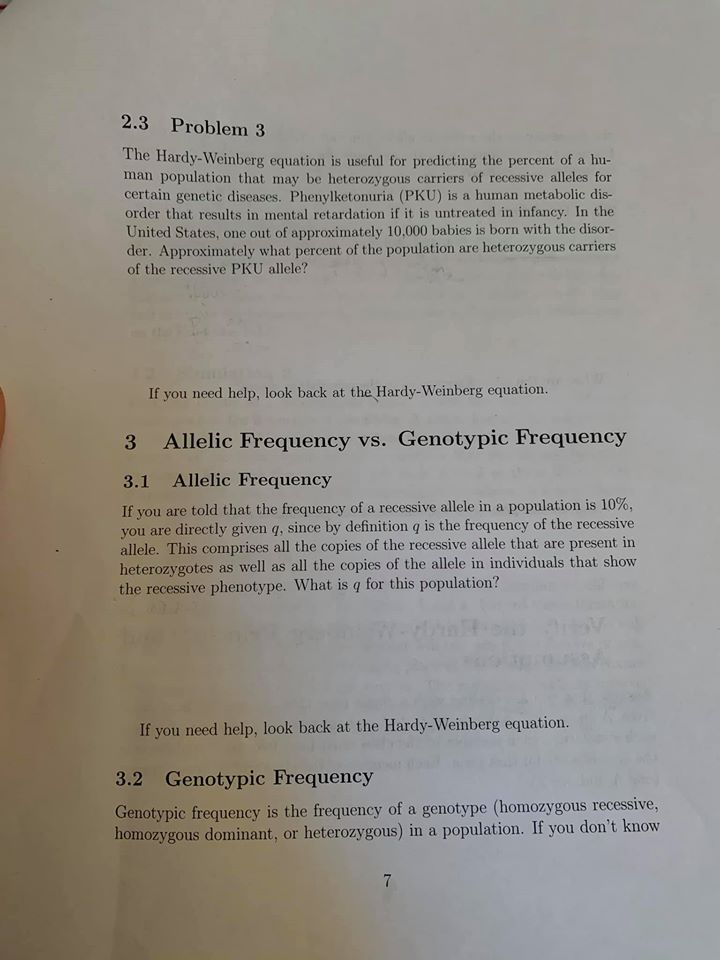2.3 Problem 3 The Hardy-Weinberg equation is useful for predicting the percent of a hu- man population that may be heterozygous carriers of recessive alleles for certain genetic diseases. Phenylketonuria (PKU) is a human metabolic dis- order that results in mental retardation if it is untreated in infancy. In the United States, one out of approximately 10.000 babies is born with the disor- der. Approximately what percent of the population are heterozygous carriers of the recessive PKU allele? If you...

• ### Which of the following is NOT true regarding Hardy-Weinberg equilibrium (HWE)?

Question 1 Which of the following is NOT true regarding Hardy-Weinberg equilibrium (HWE)? Most real species will not be at HWE at all loci within their genome If a locus has genotype frequencies consistent with HWE, then the species as a whole is not evolving If a locus has genotype frequencies consistent with HWE, then no evolution is occurring at that locus If a locus does NOT have genotype frequencies consistent with HWE, then some form of evolution is occurring at that locus Question 2 Which of...

• ### It is exceedingly rare for all the Hardy-Weinberg assumptions to be met in nature.

It is exceedingly rare for all the Hardy-Weinberg assumptions to be met in nature. Evolution is a change in allele frequencies in a population over time, so a population in Hardy-Weinberg equilibrium is not evolving. Match the following terms with the most correct statement. Each of these relates to evolutionary forces or conditions that violate the Hardy- Weinberg assumptions. (Each term only matches to one statement).  Genetic drift - Migration - Inbreeding - 4 Mutation - Natural selection - Nonrandom mating a. Does...

• ### reting Data: Hardy-Weinberg Equation 2 of 10 you use the Hardy Weinberg equation to answer questions...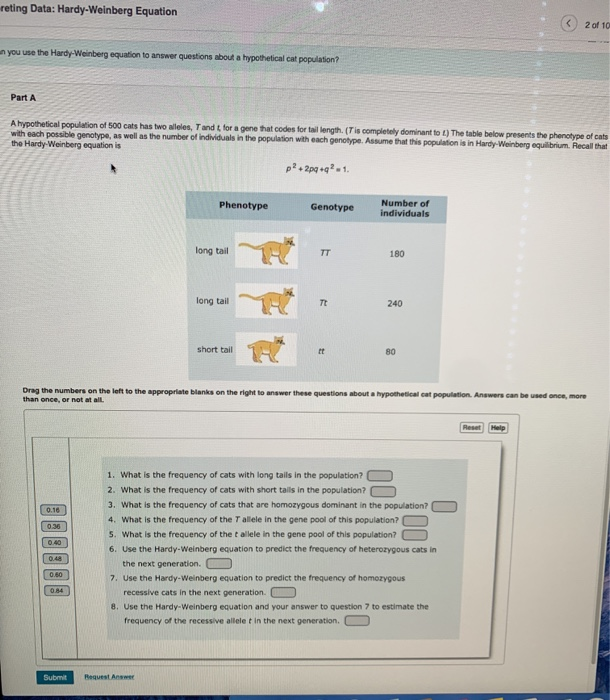reting Data: Hardy-Weinberg Equation 2 of 10 you use the Hardy Weinberg equation to answer questions about a hypotheticalcat population Part A A hypothetical population of 500 cats has two wees, Tandt for a gene that codes for tail length (Tis completely dominantot) The table below presents the phenotype of cats with each possible genotype, as well as the number of individuals in the population with each genotype. Assume that this population is in Hardy-Weinberg equilibrium Recall that the Hardy...

• ### In a population of Mendel's garden peas, the frequency of the dominant A (purple flower) allele...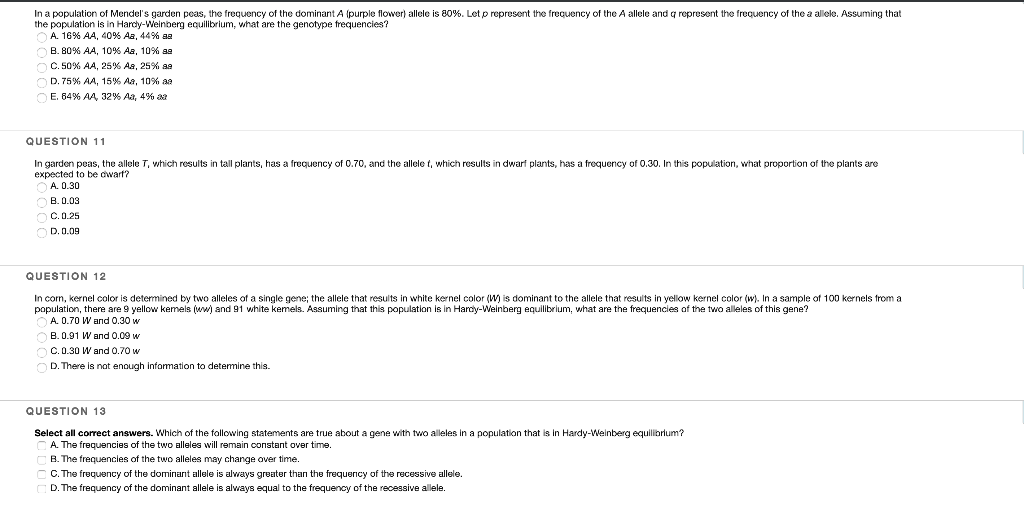In a population of Mendel's garden peas, the frequency of the dominant A (purple flower) allele is 80%. Letp represent the frequency of the A allele and q represent the frequency of the a allele. Assuming that the population is in Hardy-Weinberg equilibrium, what are the genotype frequencies? A. 16% AA, 40 % Aa, 44 % aa B. 80% AA, 10 % Aa, 10 % aa C. 50 % AA , 25 % As , 25 % aa * E....

• ### Why is it necessary for alleles to be combined randomly for the Hardy-Weinberg equation? O Random...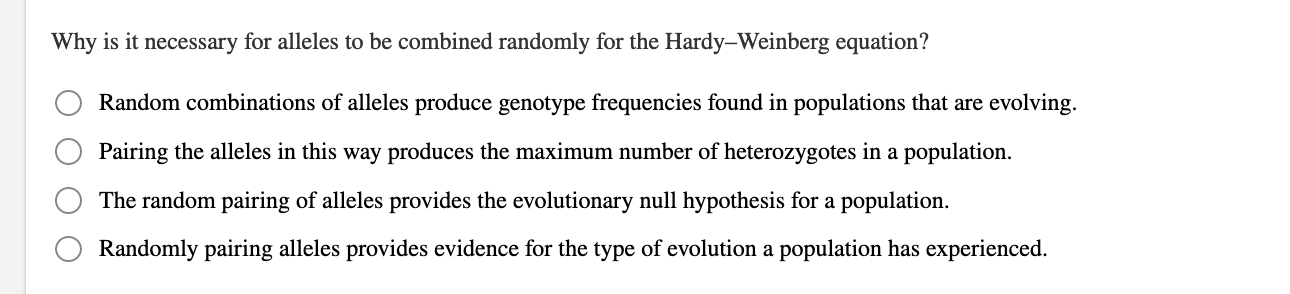Why is it necessary for alleles to be combined randomly for the Hardy-Weinberg equation? O Random combinations of alleles produce genotype frequencies found in populations that are evolving. Pairing the alleles in this way produces the maximum number of heterozygotes in a population. The random pairing of alleles provides the evolutionary null hypothesis for a population. O Randomly pairing alleles provides evidence for the type of evolution a population has experienced.

• ### 1.)If the population frequencies of two alleles at a locus are B = 0.5 and b...

1.)If the population frequencies of two alleles at a locus are B = 0.5 and b = 0.5, what is onepossible set of frequencies for the three resulting genotypes that would NOT reflect Hardy- Weinberg equilibrium? 2.)In a population that is in Hardy-Weinberg equilibrium, the frequency of the homozygous recessive genotype is 0.09. What is the frequency of individuals that are homozygous for the dominant allele? 3.)In humans, Rh-positive individuals have the Rh antigen on their red blood cells, while...

• ### 1. Fixation of Dominant Alleles Start with a population that has a gene with two alleles (A and a...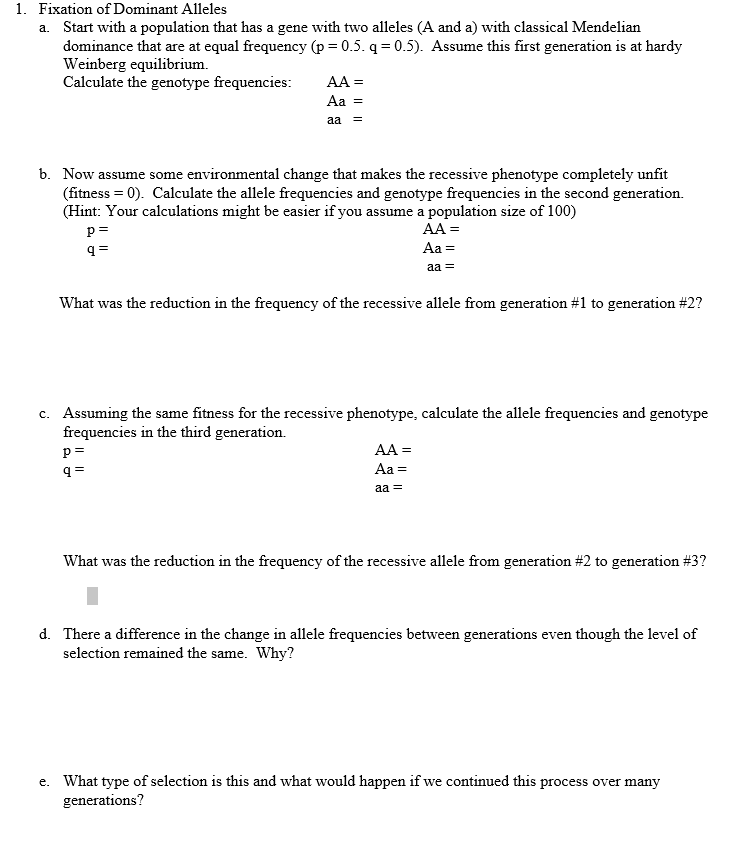1. Fixation of Dominant Alleles Start with a population that has a gene with two alleles (A and a) with classical Mendelian dominance that are at equal frequency (p0.5. q 0.5). Assume this first generation is at hardy Weinberg equilibrium. Calculate the genotype frequencies AA- a. Aa b. Now assume some environmental change that makes the recessive phenotype completely unfit (fitness- 0). Calculate the allele frequencies and genotype frequencies in the second generation. (Hint: Your calculations might be easier if...

• ### - The Hardy-Weinberg equation does not really apply to populations like our genetics class. ey not?...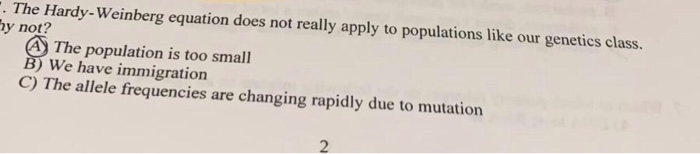- The Hardy-Weinberg equation does not really apply to populations like our genetics class. ey not? A The population is too small B) We have immigration C) The allele frequencies are changing rapidly due to mutation D) The genes are changing but not alleles E) There is strong selection on genes in our class